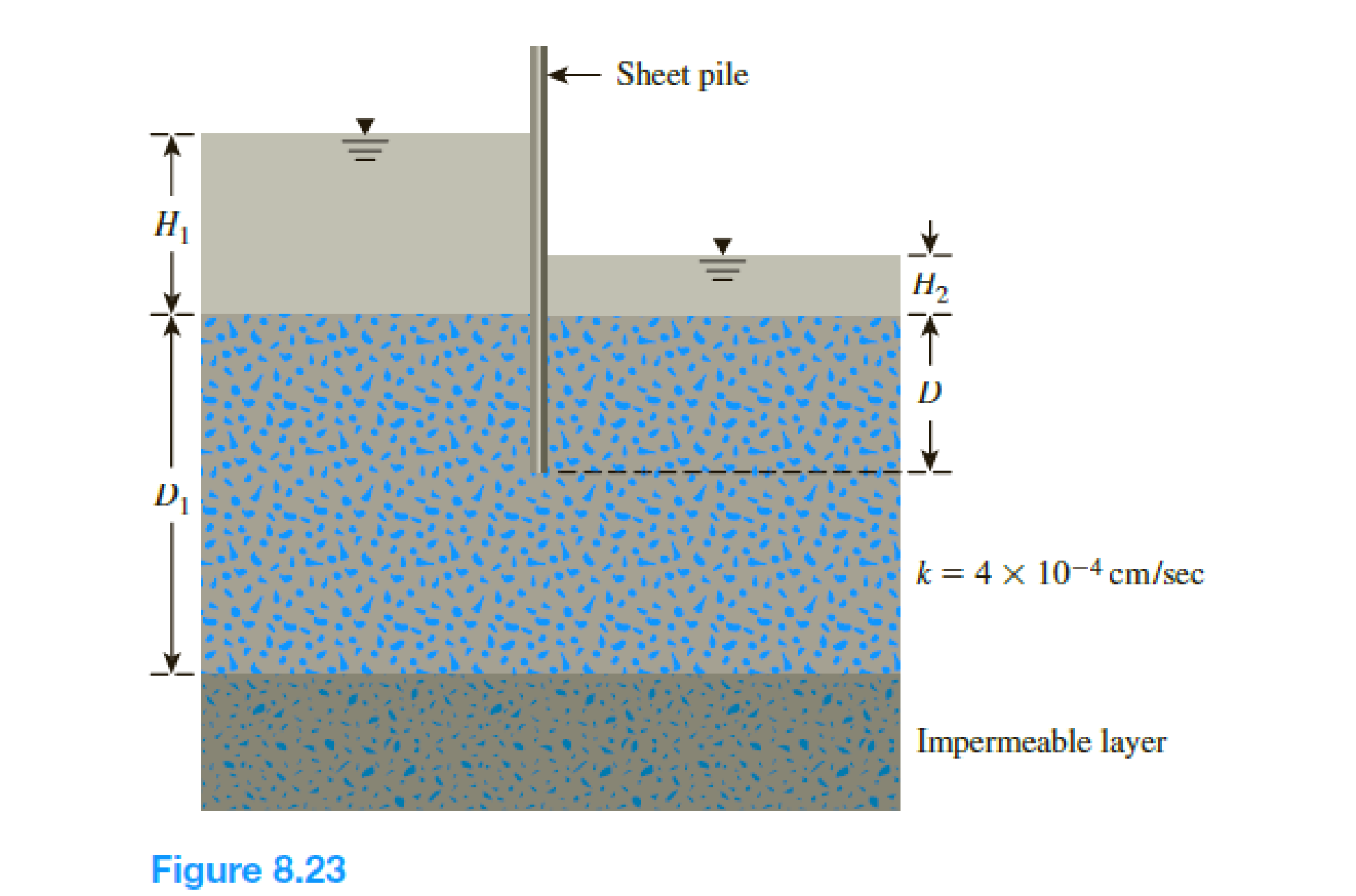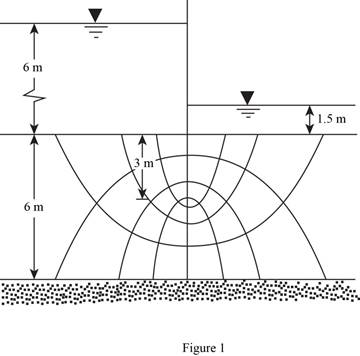Chapter 8, Problem 8.1PPrinciples of Geotechnical Enginee...

9th Edition
Braja M. Das + 1 other
ISBN: 9781305970939

Solutions

Chapter
SectionPrinciples of Geotechnical Enginee...

9th Edition
Braja M. Das + 1 other
ISBN: 9781305970939
Textbook Problem

Refer to Figure 8.23. Given: • H1 = 6m • H2 = 1.5 m • D = 3 m • D1 = 6 m Draw a flow net. Calculate the seepage loss per meter length of the sheet pile (at a right angle to the cross section shown).To determine

Draw the flow net and calculate the seepage loss per meter length of the sheet pile.

The seepage loss per meter length of the sheet pile is 77.76×102m3/m/day_.

Explanation

Given information:

The hydraulic conductivity of the permeable soil layer k is 4×104cm/sec.

The height of the water level H1 is 6 m.

The height of the water level H2 is 1.5 m.

The depth of permeable layer up to the end of sheet pile D is 3 m.

The depth of permeable layer D1 is 6 m.

Calculation:

Draw the free body diagram of the flow net for the given values as in Figure (1).Refer Figure 1.

Determine the head difference between the upstream and downstream using the relation.

H=H1H2

Substitute 6 m for H1 and 1.5 m for H2.

H=61.5=4.5m

Determine the seepage loss per meter length of the sheet pile using the relation.

q=kHNfNd

Here, Nf is the number of flow lines and Nd is the number of potential drops.

Substitute 4×104cm/sec for k, 4.5 m for H, 4 for Nf, and 8 for Nd.

q=4×104cm/sec×1m100cm×86,400sec1day×4.5×48=77.76×102m3/m/day

Therefore, the seepage loss per meter length of the sheet pile is 77.76×102m3/m/day_.

The Solution to Your Study Problems

Bartleby provides explanations to thousands of textbook problems written by our experts, many with advanced degrees!

Get Started

What is a DBMS, and what are its functions?

Database Systems: Design, Implementation, & Management

What are the basic characteristics of a NoSQL database?

Database Systems: Design, Implementation, & Management

What is a VPN? Why is it becoming more widely used?

Principles of Information Security (MindTap Course List)

Find all the answers to your study problems with bartleby.
Textbook solutions plus Q&A. Get As ASAP arrow_forward